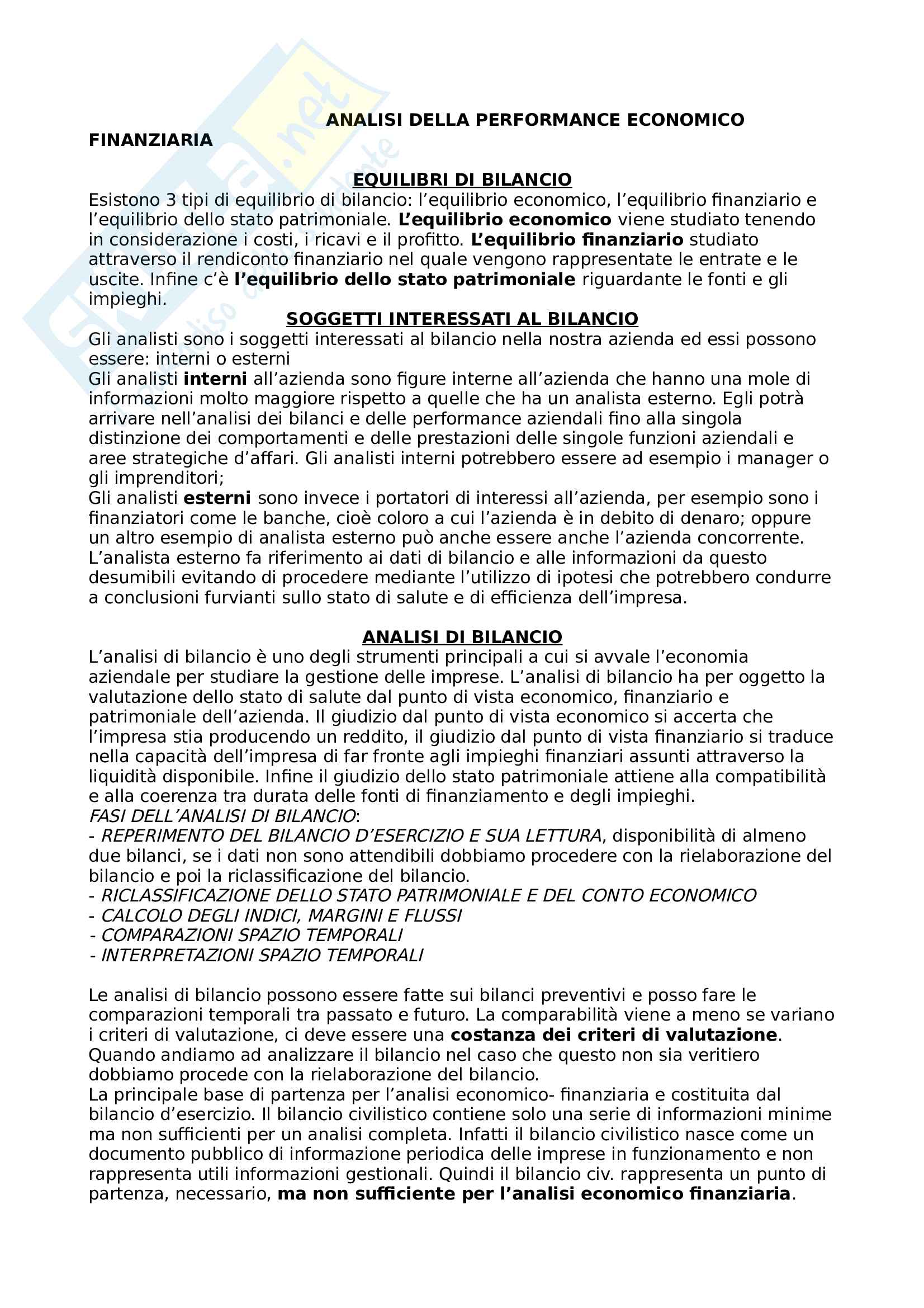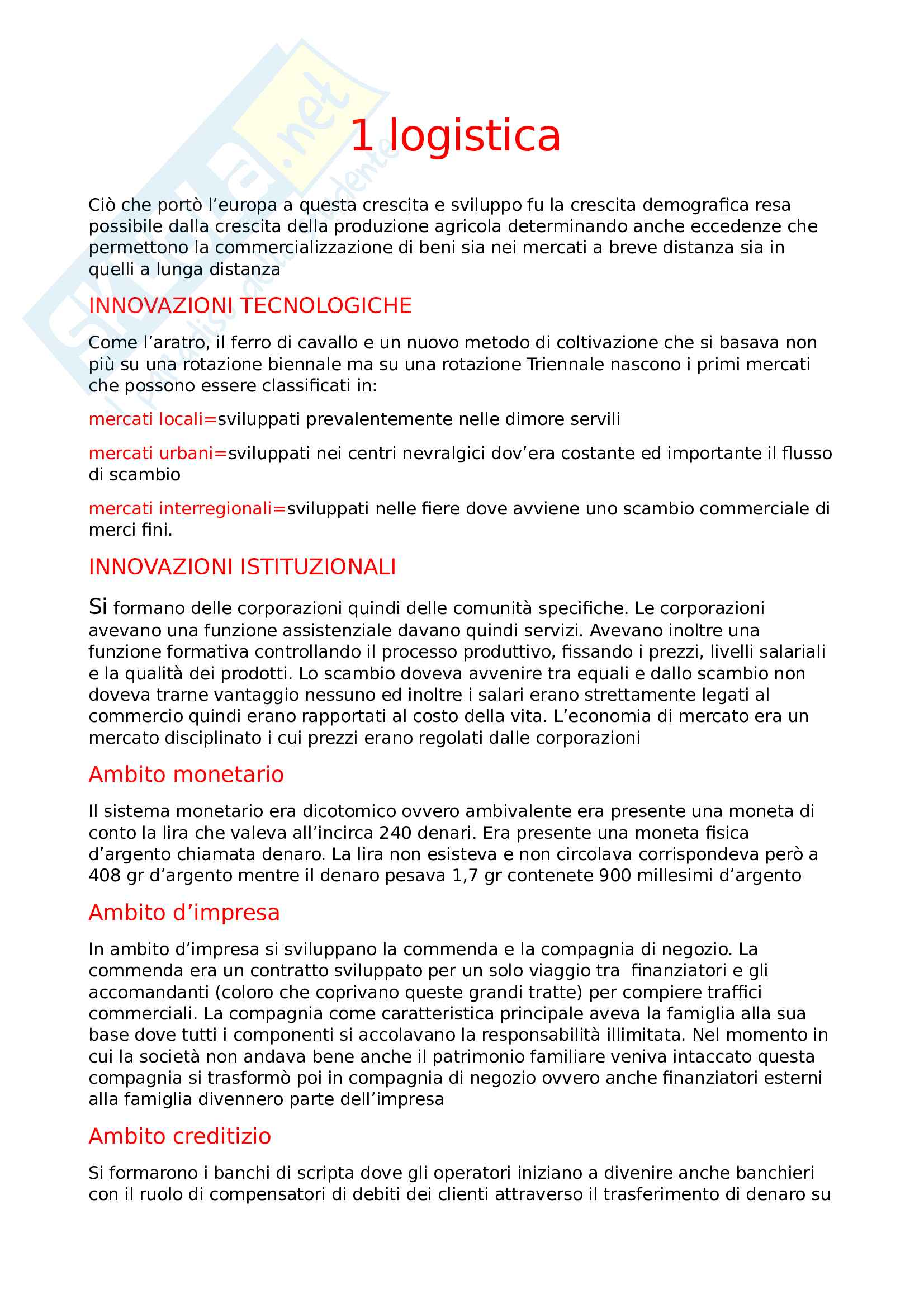Che materia stai cercando?

# Prove d'esame prof Bontempi - Econometria

Il documento riporta in lingua inglese, quattro esercitazioni d'esame della prof Maria Elena Bntempi. Il testo degli esercizi è reperibile sul suo sito personale (facilmente rintracciabile dalla pagina Unibo). Le prove riportate sono la numero 0,1,2,3.

Esame di Econometrics docente Prof. M. Bontempi

Anteprima

### ESTRATTO DOCUMENTO

PROVA 0

Through the command summ varname on STATA we are able to find out some important

1. things:

The dependent variable ahe( average hourly earnings) is not distributed as a normal variable: the

skewness is different from zero and the kurtosis higher than three. So, it is well approximated by a

t student. From the positive skewness we can state that most of the values will be located under

the right tail of the distribution.

Through the skwtest, we have a pvalue equal to zero (lower than alfa=0.05) consequently we

reject the null hypothesis in favor of the alternative one. The dependent variable is not a normal.

The 42.77% of observations are females. Through the bivariate regression model we find

2. out the coefficient of female equal to: -4.411585. It represents the difference between

women and men in terms of average hourly earnings. The pvalue is equal to 0 and the

confidential interval does not include 0, consequently we can state that this parameter is

significantly different from zero: it exist a difference between women and men, in particular,

women earns less than men(4.40 euro less on average).

The hypothesis behind answer 2 is that we have only a dummy variable in our regression

3. model without taking into consideration other explanatory variables. To answer we need a

new regression model.

Through the new multivariate regression model we find out a positive coefficient for years of

4. education, to say that one extra year of education will implies 1.80 euro more in terms of

average hourly earnings. However, the values of female coefficient is still high (-4.58) and

significantly different from zero, saying that probably we have a problem of gender

discrimination.

The new variable age has a positive coefficient but close to zero. Anyway through the t test

5. we can state that it is significantly different from zero (p value equal to zero). Looking at the

R squared (goodness of fit measure) we can say that age adds something to the model as

Rsquared has increased from 21% to 24% but note that still the 75% of the dependent

variable is unexplained!

Despite this fact, the last regression seems better than the previous two.

As the coefficient of our dummy variables are statistically different from zero we can state

6. that there are significant differences in the average hourly earnings according to the

regions. We can also test these dummy variables jointly through the f test. We take the

differences between them in terms of expected value and develop a test in which according

to our null hypothesis their coefficients are equal to zero. We find out a pvalue equal to

zero. We reject the null in favor of the alternative hypothesis: also jointly there are

differences among regions.

In this new regression one dummy variable has been dropped by STATA due to multicollinearity

problem. We have multicollinearity when one variable can be defined also in terms of another

variable. When dealing with dummy variables we always have this problem; to solve it and

compute the regression one variable must be dropped.

May be interested to note that the coefficients of female is more or less the same, no matter what

is the number of explanatory variables and/or dummy variables. So, it can be defined robust.

We cannot compare the log level model with the level level model because we have two

7. different dependent variables. 1

PROVA 1

We are dealing with a cross sectional data. Through the command descr we obtain some

Sav: annual savings, $- Inc: annual income,$

- Size: family size

- Educ: years of education, household head

- Age: age of household head

- Black: =1 if household head is black, 0= otherwise

- Cons: annual consumption \$

-

as well as the number of observations which is equal to 1000.

The command summ sav, d allows us to say that the dependent variable has not a normal

distribution. Indeed, it has a skewness different from zero and a kurtosis different from three. This

result is confirmed also by the sktest, which shows a pvalue equal to zero. (with Alfa equal to 5%)

In particular, the skewness is positive saying that most of the values of y are situated under the

right tail of the distribution. This result is suggested also by the mean, which is significantly higher

than the median. As regard the kurtosis, it is higher than three telling us that our dependent

variable is well approximated by a t student.

From the correlation command on STATA, we can see that all the explanatory variables (except for

consumption) are positively correlated with Y.

By comparing the two regression models, we see that the coefficient of income (beta1)

2. becomes statistically insignificant. Indeed, in the simple regression model it has a pvalue

equal to 0.012 but this is not still true in the multiple regression model where the p value is

0.129. Moreover, we can see that also all the other explanatory variables are not

statistically different from zero.

The adjusted R squared is low in both the simple and the multiple regression model, in particular it

decreases from the first to the second. This fact suggests that all the demographic variables

together with income are not useful in explaining Y (97% is unexplained!)

2 possibile risposta alla multicollinearity:

From the correlation between all the explanatory variables, we are able to see that between

income and education there is a high (positive) correlation equal to 46%. We can test these two

variables jointly through the F test in order to verify the presence of multicollinearity. The pvalue of

the F test is lower that 5% saying that we must reject H0 in favor of H1. This result is not

consistent with the t test, which suggest the opposite.

So, there is multicollinearity between income and education: we must cut one of this two in order

to study the regression. 2

PAGINE

5

PESO

25.13 KB

AUTORE

PUBBLICATO

+1 anno fa

DETTAGLI
Esame: Econometrics
Corso di laurea: Corso di laurea magistrale in economia e management
SSD:
Università: Bologna - Unibo
A.A.: 2017-2018

I contenuti di questa pagina costituiscono rielaborazioni personali del Publisher .Giulia11 di informazioni apprese con la frequenza delle lezioni di Econometrics e studio autonomo di eventuali libri di riferimento in preparazione dell'esame finale o della tesi. Non devono intendersi come materiale ufficiale dell'università Bologna - Unibo o del prof Bontempi Maria Elena.

Acquista con carta o conto PayPal

Scarica il file tutte le volte che vuoi

Paga con un conto PayPal per usufruire della garanzia Soddisfatto o rimborsato

RecensioniTi è piaciuto questo appunto? Valutalo!

## Altri appunti di Corso di laurea magistrale in economia e managementAppunto

### Analisi della performance economico finanziariaAppunto